﻿ 函数型线性判别分析

# 函数型线性判别分析Linear Discriminant Analysis for Functional Data

Abstract: In this paper, functional linear discriminant analysis method is proposed for the classification problem of input as functional data. By introducing the functional norm to measure the distance within-class and between-class, an optimization model of functional linear discriminant analysis is constructed. Furthermore, by using the basis function method to transform the infinite dimensional function space into a finite dimensional optimization model, then this model is easy to solve. Since the data is functional, the first derivative or the second derivative of the function can be found. The classification result can be further improved by using the data after the derivative. Finally, the numerical experiments show the feasibility and effectiveness of the functional linear discriminant analysis method.

1. 引言

2. 相关工作

${S}_{w}=\underset{x\in {X}_{1}}{\sum }\left(x-{\mu }_{1}\right){\left(x-{\mu }_{1}\right)}^{T}+\underset{x\in {X}_{2}}{\sum }\left(x-{\mu }_{2}\right){\left(x-{\mu }_{2}\right)}^{T}$ (1)

${S}_{b}=\left({\mu }_{1}-{\mu }_{2}\right){\left({\mu }_{1}-{\mu }_{2}\right)}^{T}$ (2)

$\mathrm{max}J=\frac{{w}^{T}{S}_{b}w}{{w}^{T}{S}_{w}w}$ (3)

3. 函数型线性判别分析

$\mathrm{max}J=\frac{{‖\int w\left(t\right){\mu }_{1}\left(t\right)-\int w\left(t\right){\mu }_{2}\left(t\right)‖}_{2}^{2}}{Var\int w\left(t\right){x}_{1}\left(t\right)+Var\int w\left(t\right){x}_{2}\left(t\right)}$ (4)

${\stackrel{^}{x}}_{j}\left(t\right)=\underset{k=1}{\overset{K}{\sum }}{c}_{jk}{\varphi }_{k}\left(t\right)$ (5)

${\stackrel{^}{x}}_{j}\left(t\right)={C}_{j}^{T}\Phi ={\Phi }^{T}{C}_{j}$ (6)

$\begin{array}{c}\mathrm{var}\int w\left(t\right){x}_{j1}\left(t\right)={N}^{-1}\int w\left(t\right){x}_{j1}\left(t\right)\int w\left(t\right){x}_{j1}\left(t\right)\\ ={N}^{-1}\int {\text{d}}^{T}\Phi {\Phi }^{T}\stackrel{¯}{C}\int {\left({\text{d}}^{T}\Phi {\Phi }^{T}\stackrel{¯}{C}\right)}^{T}\\ ={N}^{-1}{\text{d}}^{T}\int \Phi {\Phi }^{T}\stackrel{¯}{C}{\stackrel{¯}{C}}^{T}\int \Phi {\Phi }^{T}\text{d}\\ ={\text{d}}^{T}J{V}_{0}J\text{d}\end{array}$ (7)

$\begin{array}{c}\mathrm{var}\int w\left(t\right){x}_{j2}\left(t\right)={N}^{-1}\int w{x}_{j2}\int w{x}_{j2}\\ ={N}^{-1}\int {\text{d}}^{T}\Phi {\Phi }^{T}\stackrel{^}{C}\int {\left({\text{d}}^{T}\Phi {\Phi }^{T}\stackrel{^}{C}\right)}^{T}\\ ={N}^{-1}{\text{d}}^{T}\int \Phi {\Phi }^{T}\stackrel{^}{C}{\stackrel{^}{C}}^{T}\int \Phi {\Phi }^{T}\text{d}\\ ={\text{d}}^{T}J{V}_{1}J\text{d}\end{array}$ (8)

$\begin{array}{c}{‖\int w\left(t\right){\mu }_{1}\left(t\right)-\int w\left(t\right){\mu }_{2}\left(t\right)‖}_{2}^{2}=\int w\left(t\right)\left({\mu }_{1}\left(t\right)-{\mu }_{2}\left(t\right)\right)\text{d}t\int w\left(t\right)\left({\mu }_{1}\left(t\right)-{\mu }_{2}\left(t\right)\right)\text{d}t\\ =\int {\text{d}}^{T}\Phi {\Phi }^{T}m\int {\left({\text{d}}^{T}\Phi {\Phi }^{T}m\right)}^{T}\\ ={d}^{T}\int \Phi {\Phi }^{T}m{m}^{T}\int \Phi {\Phi }^{T}\text{d}\\ ={d}^{T}JVJ\text{d}\end{array}$ (9)

$\mathrm{max}J\left(d\right)=\frac{{d}^{T}JVJd}{{d}^{T}J\left({V}_{0}+{V}_{1}\right)Jd}$ (10)

$\begin{array}{cc}\underset{d}{\mathrm{min}}& -{d}^{T}JVJd\\ \text{s}\text{.t}& {d}^{T}J\left({V}_{0}+{V}_{1}\right)Jd=1\end{array}$ (11)

$L\left(d,\lambda \right)=-{d}^{T}JVJd+\lambda \left[{d}^{T}J\left({V}_{0}+{V}_{1}\right)Jd-1\right]$ (12)

$\frac{\partial L}{\partial d}=-2JVJd+2\lambda J\left({V}_{0}+{V}_{1}\right)Jd=0$ (13)

$JVJd=\lambda J\left({V}_{0}+{V}_{1}\right)Jd$ (14)

$L=J\left({V}_{0}+{V}_{1}\right)J$ ，即求 ${L}^{-1}JVJd=\lambda d$ 的一般特征值问题。

1) 选择基函数，求解样本函数的系数 $\stackrel{¯}{C},\stackrel{^}{C}$

2) 计算协方差矩阵 ${V}_{0},{V}_{1},V$ 和基矩阵 $J$

3) 计算(14)式得出 $\lambda$

4) 代入 ${f}_{j}=\int wx=\int w\left(t\right){x}_{j}\left(t\right)\text{d}t$ 并计算 $\mathrm{min}\text{\hspace{0.17em}}{‖{f}_{j}-{\mu }_{i}\left(t\right)‖}^{2}$

4. 数值实验

4.1. 人工数据的数值实验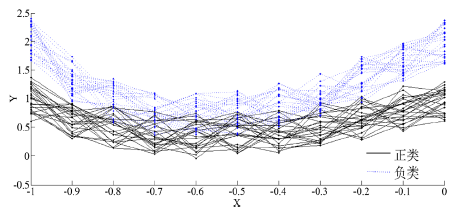(a)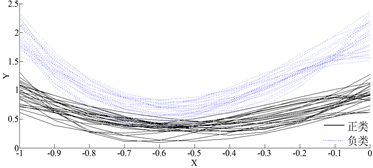(b)

Figure 1. (a) Curves of 50 different types of artificial data sets, (b) Curves fitted by basis function methodFigure 2. Classification of 50 curves using functional linear discriminant analysis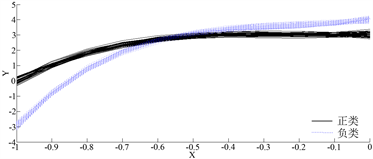(a)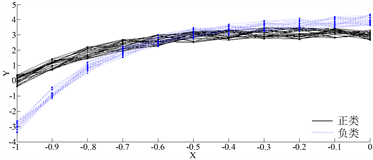(b)

Figure 3. (a) Curves of 50 different types of artificial data sets, (b) Curve fitted by basis function method

4.2. Spectrometric数据集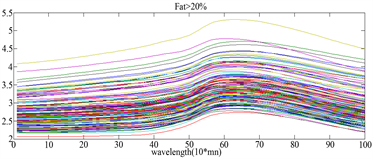(a)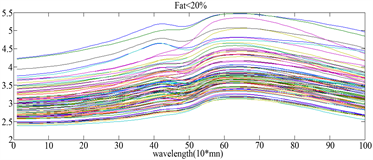(b)

Figure 4. First-order derivation of spectrometric data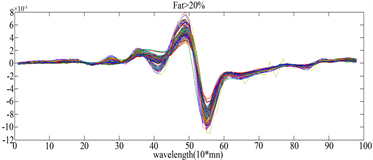(a)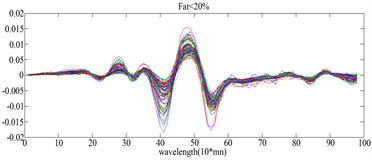(b)

Figure 5. Second-order derivation of spectrometric data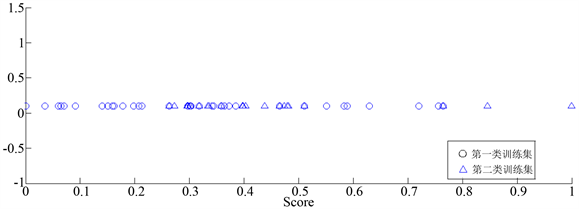Figure 6. Take 20% of the spectrometric data after the first-order derivation classification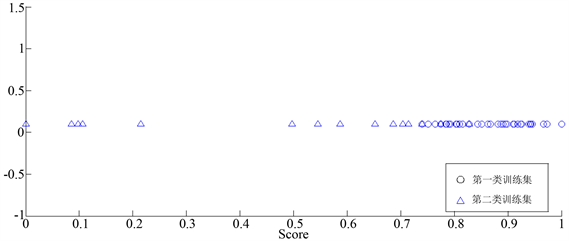Figure 7. Take 20% of the spectrometric data after the second-order derivation classification

5. 结论

 周志华. 机器学习[M]. 北京: 清华大学出版社, 2016.

 张学工. 关于统计学习理论与支持向量机[J]. 自动化学报, 2000, 26(1): 36-46.

 平源. 基于支持向量机的聚类及文本分类研究[D]: [博士学位论文]. 北京: 北京邮电大学, 2012.

 田盛丰, 黄厚宽. 基于支持向量机的手写体相似字识别[J]. 中文信息学报, 2000, 14(3): 37-41.

 邹建法, 王国胤, 龚勋. 基于增强Gabor特征和直接分步线性判别分析的人脸识别[J]. 模式识别与人工智能, 2010, 23(4): 477-482.

 Fisher, R.A. (1936) The Use of Multiple Measurements in Taxonomic Problems. Annals of Human Genetics, 7, 179-188.
https://doi.org/10.1111/j.1469-1809.1936.tb02137.x

 Belhumeur, P.N., Hespanha, J.P. and Kriegman, D.J. (1997) Eigenfaces versus Fisherfaces: Recognition Using Class Specific Linear Projection. IEEE Transactions on Pattern Analysis and Machine Intelligence, 19, 711-720.
https://doi.org/10.1109/34.598228

 Rezzi, S., Giani, I., Heberger, K., et al. (2007) Classification of Gilthead Sea Bream (Sparus aurata) from 1H NMR Lipid Profiling Combined with Principal Component and Linear Discriminant Analysis. Journal of Agricultural and Food Chemistry, 55, 9963-9968.
https://doi.org/10.1021/jf070736g

 张新新, 李雨, 等. 主成分-线性判别分析在中药药性识别中的应用[J]. 山东大学学报, 2012, 50(1): 143-146.

 栗科峰, 卢金燕, 等. 基于子图分割与多类支持向量机的人脸识别方法[J]. 科技通报, 2018(8): 1001-7119.

 Li, M. and Yuan, B. (2005) 2D-LDA: A Statistical Linear Discriminant Analysis for Image Matrix. Pattern Recognition Letters, 26, 527-532.
https://doi.org/10.1016/j.patrec.2004.09.007

 Ramsay, J.O. (1982) When the Data Are Functions. Psychometrika, 47, 379-396.
https://doi.org/10.1007/BF02293704

 Ramsay, J.O. and Dalzell, C.J. (1991) Some Tools for Functional Data Analysis. Journal of the Royal Statistical Society, Series B, 53, 539-572.
https://doi.org/10.1111/j.2517-6161.1991.tb01844.x

 Ramsay, J.O. and Silverman, B.W. (1997) Functional Data Analysis. Springer-Verlag, New York.
https://doi.org/10.1007/978-1-4757-7107-7

Top